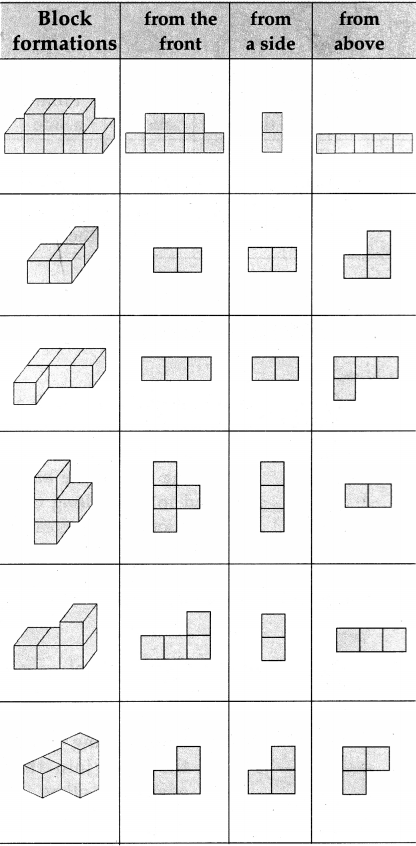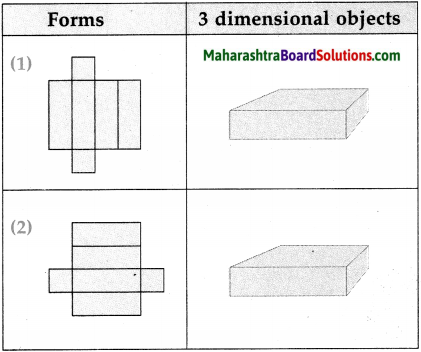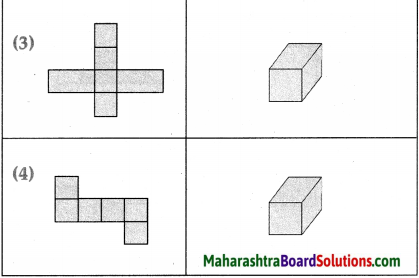# Maharashtra Board Class 5 Maths Solutions Chapter 13 Three Dimensional Objects and Nets Problem Set 51

Balbharti Maharashtra Board Class 5 Maths Solutions Chapter 13 Three Dimensional Objects and Nets Problem Set 51 Textbook Exercise Important Questions and Answers.

## Maharashtra State Board Class 5 Maths Solutions Chapter 13 Three Dimensional Objects and Nets Problem Set 51

Question 1.
The first column shows a structure made of blocks. The other columns show different views of the structure in two dimensions. Say whether each view is from the front, from a side or from above.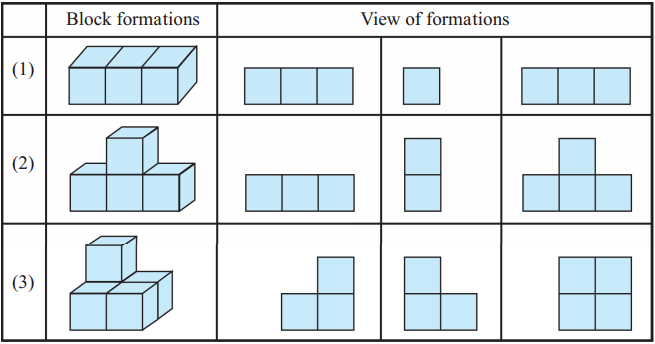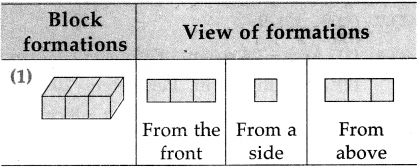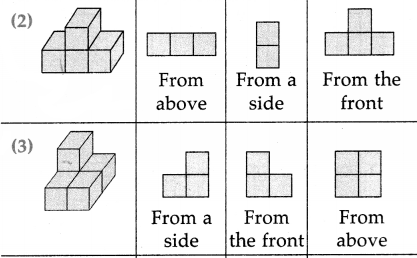Question 2.
Draw three pictures of each of these three-dimensional objects – a table, a chair and a water bottle as viewed from the front, from a side and from above.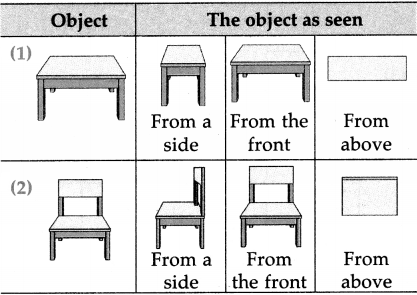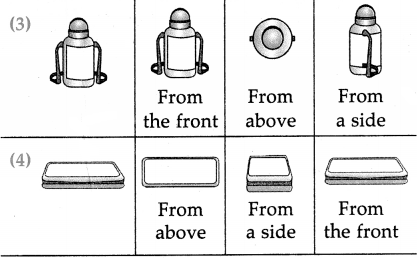Nets
Last year we saw that cutting some edges of a box and laying it out flat gives us the net from which it was made.
The two dimensional shape from which a three dimensional object can be made by folding is called the ‘net’ of that object.

1. By folding the cardboard shown below, along the lines shown in it, we get a three dimensional object (box). In this shape, all surfaces are square.
An object of this shape is called a cube.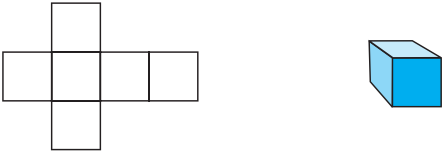2. The net of another cardboard box is shown in the figure below. By folding along the lines in this net and joining the edges to each other, we can see that a three dimensional box is formed. The surfaces of this box are rectangular in shape.
An object of this shape is called a cuboid.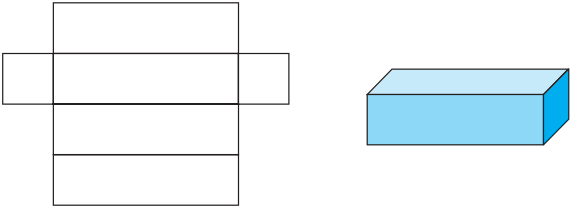Activity :
Draw the nets shown below on card sheet. Cut out the shapes and find out the shapes of the boxes they form.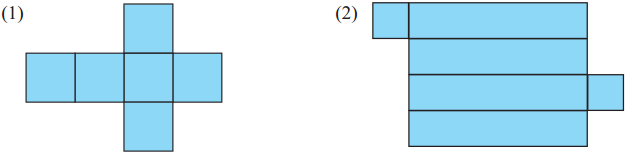A five-square net (Pentomino)

In the figure alongside, five squares of the same size are placed together with their sides joined.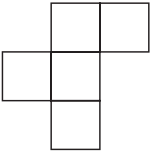Such an arrangement of five squares is called a ‘five-square net’ or a ‘pentomino’.

By folding along the edges of such a five-square net, an open box is formed.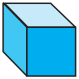Activity :
Some five-square nets are given below. Draw these nets on a card sheet. Make open boxes from these nets.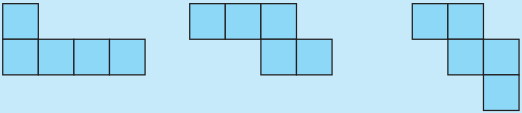Try to find out other five-square nets that can be used to make open boxes.A riddle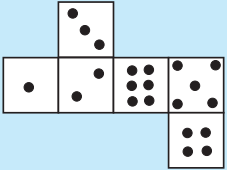The net of a cube-shaped dice is given alongside. If a dice is made of this net, which of the following shapes will it definitely not resemble?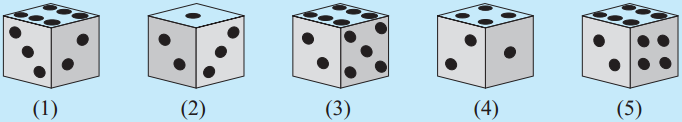Chapter 12 Perimeter and Area Problem Set 49 Additional Important Questions and Answers

Question 1.
Draw the pictures of each of these three dimensional objects – Mobile, Oil tin as viewed from the front, from a side and from above.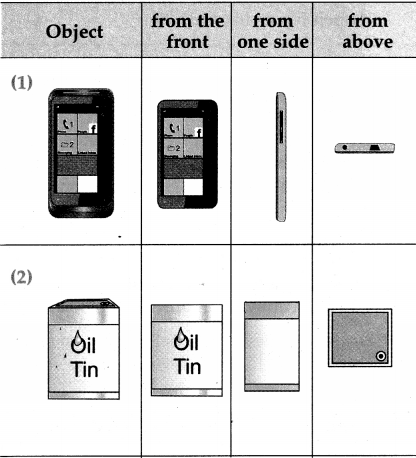Question 2.
The three dimensional figure of block formation is shown in the figure along side. Draw as view from the front, from a side and from above (fig. drawn in answer part)